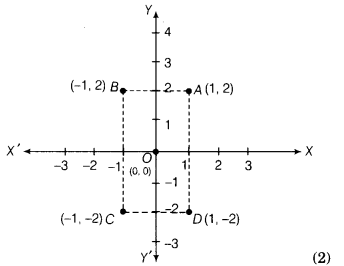# (i)Plot these points on a single sheet of a graph paper.(ii)Are they at equal distance from the well?

Four friends Ashwani, Tarun, Shiva and Deepak are standing at the points A, B, C and D reference to a well situated at the origin (0, 0) with the respective coordinates (1, 2), (-1,2), (-1,-2) and (1,-2).
(i) Plot these points on a single sheet of a graph paper.
(ii) Are they at equal distance from the well?

(i) The points A (1,2), B(-1,2), C(-1, -2) and D(1, -2) can be plotted as shown below(ii) Joining all these points on a graph paper, we get a rectangle.
We know that diagonals of a rectangle are equal and bisect each other.
∴ OA = OB = OC = OD
Hence, all four friends are at equal distance from the well.